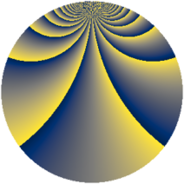# Properties

 Label 1600.2.bmLevel $1600$ Weight $2$ Character orbit 1600.bm Rep. character $\chi_{1600}(101,\cdot)$ Character field $\Q(\zeta_{16})$ Dimension $1192$ Sturm bound $480$

# Related objects

## Defining parameters

 Level: $$N$$ $$=$$ $$1600 = 2^{6} \cdot 5^{2}$$ Weight: $$k$$ $$=$$ $$2$$ Character orbit: $$[\chi]$$ $$=$$ 1600.bm (of order $$16$$ and degree $$8$$) Character conductor: $$\operatorname{cond}(\chi)$$ $$=$$ $$64$$ Character field: $$\Q(\zeta_{16})$$ Sturm bound: $$480$$

## Dimensions

The following table gives the dimensions of various subspaces of $$M_{2}(1600, [\chi])$$.

Total New Old
Modular forms 1968 1240 728
Cusp forms 1872 1192 680
Eisenstein series 96 48 48

## Trace form

 $$1192q + 8q^{2} + 8q^{3} + 8q^{4} - 24q^{6} + 8q^{7} + 8q^{8} + 8q^{9} + O(q^{10})$$ $$1192q + 8q^{2} + 8q^{3} + 8q^{4} - 24q^{6} + 8q^{7} + 8q^{8} + 8q^{9} - 24q^{11} + 8q^{12} + 8q^{13} + 8q^{14} - 24q^{16} + 8q^{17} + 8q^{18} + 8q^{19} - 24q^{21} + 16q^{22} + 8q^{23} + 48q^{24} - 64q^{26} + 8q^{27} + 48q^{28} + 8q^{29} + 48q^{32} + 48q^{34} - 104q^{36} + 8q^{37} + 48q^{38} + 8q^{39} - 24q^{41} + 48q^{42} + 8q^{43} + 16q^{44} - 24q^{46} + 8q^{47} + 8q^{48} + 8q^{49} - 56q^{51} - 40q^{52} + 8q^{53} + 72q^{54} - 128q^{56} + 8q^{57} - 64q^{58} - 56q^{59} - 24q^{61} - 56q^{62} + 96q^{63} + 104q^{64} + 56q^{66} + 88q^{67} - 40q^{68} + 8q^{69} - 88q^{71} - 64q^{72} + 8q^{73} - 96q^{74} + 40q^{76} + 8q^{77} - 16q^{78} - 24q^{79} - 24q^{81} - 72q^{82} + 8q^{83} - 104q^{84} + 80q^{86} + 8q^{87} + 88q^{88} + 8q^{89} - 24q^{91} - 144q^{92} - 16q^{93} + 232q^{94} + 112q^{96} + 144q^{98} + 80q^{99} + O(q^{100})$$

## Decomposition of $$S_{2}^{\mathrm{new}}(1600, [\chi])$$ into newform subspaces

The newforms in this space have not yet been added to the LMFDB.

## Decomposition of $$S_{2}^{\mathrm{old}}(1600, [\chi])$$ into lower level spaces

$$S_{2}^{\mathrm{old}}(1600, [\chi]) \cong$$ $$S_{2}^{\mathrm{new}}(64, [\chi])$$$$^{\oplus 3}$$$$\oplus$$$$S_{2}^{\mathrm{new}}(320, [\chi])$$$$^{\oplus 2}$$